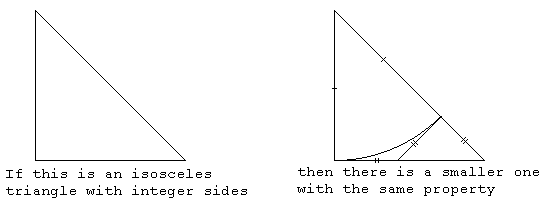Most, but perhaps not all, of us know that the square root of 2 can never be written exactly as a fraction. In other words,

• $\sqrt{2}$ is never a ratio of whole numbers,
How can we show this? Here are four proofs.

## Algebra

The usual way is as follows.

• Suppose $\sqrt{2}$ can be written as a fraction:
• $\sqrt{2}=a/b$
• Then $2=a^2/b^2$
• Then $2b^2=a^2$
• Then $a^2$ is even
• Hence $a$ is even
• (This takes a small proof of its own.)
• So we can write a=2k
• Hence $2b^2=(2k)^2=4k^2$
• So $b^2=2k^2$
• So now $b^2$ is even
• Hence $b$ is even
• So now we can reduce $\frac{a}{b}$ to a smaller fraction.
• Lather, rinse, repeat, as they say.
• Following this process we can get smaller and smaller fractions, each of which is exactly the square root of two. Clearly this is nonsense.
• The argument is sound, so the premise must be false.
• Thus we can never write $\sqrt{2}$ as $\frac{a}{b}$

## Geometry

Suppose we can draw a square that has integral sides and an integral diagonal. Using Pythagoras' theorem we can see that this is equivalent to saying that $\sqrt{2}$ is rational.

Consider the following diagram:By Pythagoras we know that if the sides are $a$ and the diagonal is $b$ then $2a^2=b^2.$ This diagram shows that given such a pair, $a,b,$ we can find a smaller pair. (Exercise for the reader: show that $a$ and $b$ in the smaller pair are still integers.)

Repeat.

Eventually we run out of numbers and thus complete our proof by contradiction

## Algebra, again

 Exercises for the diligent reader: Show that $(2a-b)^2=2(b-a)^2$ Show that b-a < a what's the relationship between this proof and the previous geometrical one?
Suppose $b^2=2a^2$ where $a,b$ are integers and as small as possible. Then a little algebra shows that $(2a-b)^2=2(b-a)^2$ so that $(b-a,2a-b)$ is a new pair of integers with the same property. But $b-a\lt~a$ and so we've got a smaller pair of integers whose ratio is $\sqrt{2},$ contrary to our original decision to take the smallest pair.

## Continued Fractions

 Another exercise for the diligent reader: Show that for every number of the form $a/b,$ its continued fraction will terminate.
Every rational number has a finite continued fraction. We show now that the continued fraction for $1+\sqrt{2}$ does not terminate. That means $1+\sqrt{2}$ is irrational, and hence $\sqrt{2}$ is irrational.

To compute the continued fraction we separate the target number into its integer part and the fractional part

• $T=1+\sqrt{2}=2+\epsilon$ where $\epsilon=\sqrt{2}-1$
$\epsilon$ is between 0 and 1 as required, so we can take its reciprocal, giving $\frac{1}{\sqrt{2}-1}$ which after rationalising the denominator is $1+\sqrt{2}.$ That's what we started with, so the continued fraction for $1+\sqrt{2}$ is:

• $\LARGE{1+\sqrt{2}=2+\frac{1}{2+\frac{1}{2+\frac{1}{2+\frac{1}{2+...}}}}}$
Thus the continued fraction for $1+\sqrt{2}$ is [2;2,2,2,2,...] which does not terminate. Hence $1+\sqrt{2}$ is irrational, and so $\sqrt{2}$ is irrational.

CategoryMaths